# Main Supervised Regression Learning Algorithms

######Supervised Learning is a branch of Machine Learning, characterized by the making of an algorithm which learns to map an input to a particular output, using a labeled training dataset. In the previous article, we looked at the basics of supervised machine learning and one of it’s branches: regression. Below, we will dive into the main algorithms used in supervised regression learning and a few applications.

### Main regression algorithms

Linear Regression

Linear regression allows to predict a dependent variable value (y) based on one or more given independent variables (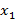,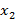,…). Hence, this regression technique establishes a linear relationship between x (the input) and y (the output).

a. Simple linear regression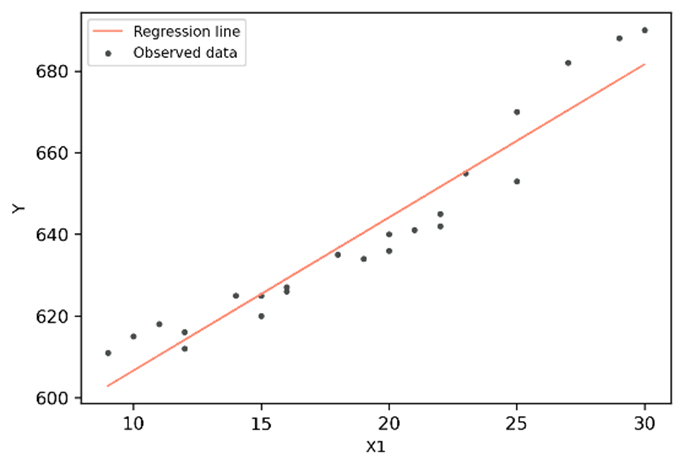In this case, the regression model will establish a linear relationship between a single independent variable (x) and a continuous dependent variable (y). Thus, the model needs to start off with a hypothesis function of the following form:​​​​​​​​​​​​

​​​​​​​​​​​​​​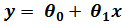In this case,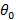​​​​​​​ corresponds to the intercept, while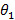corresponds to the coefficient of x or, in other words, the slope. It is expected, in the case of supervised learning, that the values for x and y will be given, while training the model. While doing so , the model will fit the best line to predict the value of y for a given input value x. To do so, it needs to find the bestandvalues, by iterating through the training dataset. To determine the optimalandvalues and establish how well our model is fitting the values, we can establish some evaluation metrics. The most popular evaluation metrics for determining performance of regression models is the Root Mean Squared Error, or RMSE, which corresponds to the root of the mean of the squared errors.
The formula is given below.

​​​​​​​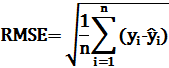​​​​​​​​​​​​​​

In this formula:

• n is the total number of data points
•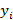is the actual output value
•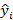is the predicted output value

Once the optimal values have been identified, the model should have established the linear relationship with the least error or, in other words, with a minimized RMSE.

Other evaluation metrics like the Mean Squared Error (MSE), the Mean Absolute Error (MAE) or the coefficient of determination (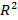) can also used.

Simple linear regression is the most common model used in finance. Indeed, it can be used for portfolio management, asset valuation or optimization. For instance, it can be used to forecast returns and the operational performances of a business. However, there are some cases in which the target or dependent variable is not impacted only by a single predictor. Therefore, we would need a model to fit these more elaborate cases: we would use multiple linear regression.​​​​​​​

b. Multiple linear regression

In some cases, the output (y) we are trying to predict depends on more than one variable. Thus a more elaborate model, that takes into account this higher dimensionality, is required. This is what we call multiple linear regression. Using more independent variables can actually improve the accuracy of the model, as long as the variables are relevant to the problem . For instance, a multilinear regression model based on three independent variables would follow a function of the following format: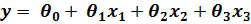In a similar fashion as for simple linear regression , evaluation metrics can be used to determine the best fit.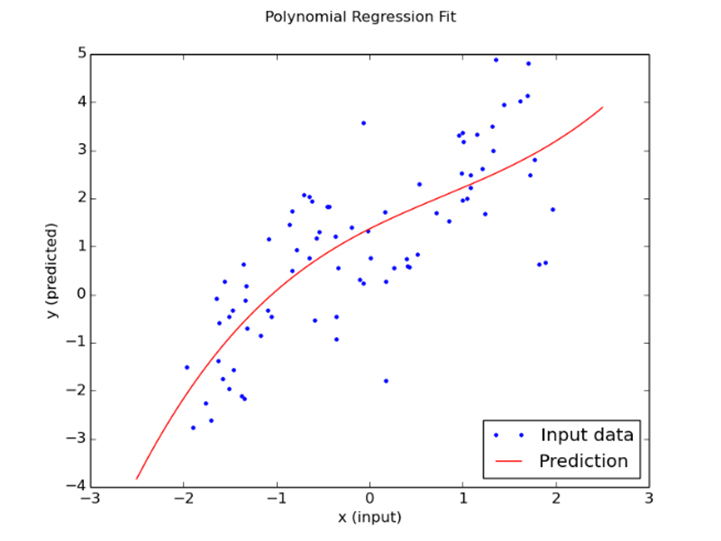c. Polynomial Regression

Polynomial regression can be considered a special case of multilinear regression, in which the data distribution is more complex than a linear one. In other words, the relationship between the dependent variable x and the independent variable y is modelled as the nth degree polynomial in x. This algorithm will generate a curve to fit non-linear data. For instance, a polynomial regression could follow the following function: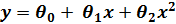​​​​​​​Poisson Regression

A second regression algorithm is the Poisson Regression. This is a special type of regression, in which the response variable, y, needs to consist of count data. In other words, this variable needs to be integers 0 or greater, and thus cannot contain negative values. Before a Poisson regression can be conducted, it needs to be verified that the observations are independent of one another and that the distribution of counts follows a Poisson distribution.

​​​​​​​Support Vector Regression

Support Vector Regression, or SVR, is a regression algorithm that works both for linear and non-linear regressions. It works on the principle of the Support Vector Machine and is used to predict continuous ordered variables. In contrast with simple linear regression, where the goal is to minimize the error rate, in SVR, the idea is to fit the error inside a determined threshold and to maximize a margin between classes. In other words, SVR tries to approximate the best value within a certain margin, that is known as the ε-tube.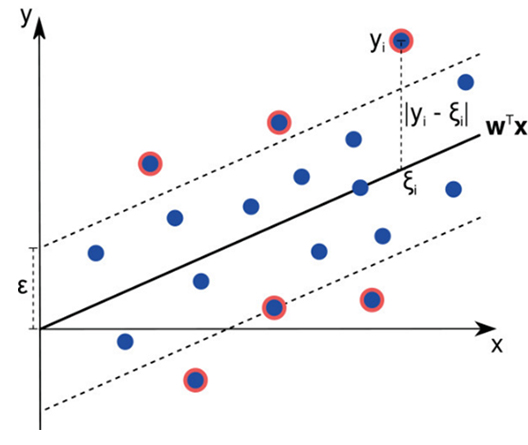​​​​​​​On the plot to the right, the full black line corresponds to the hyperplane, which helps to predict the target value. On the other hand, the dotted lines correspond to the boundary lines, drawn at a distance ε of the hyperplane. Ideally, the objective of SVR is to position the hyperplane is such a way that it separates the independent variable of one class from another. SVR are amongst the most widely used techniques for stock price predictions, as these algorithms are now able to anticipate market movements consistently and therefore estimate the future asset values, such as stock prices. Similarly, as SVR is particularly effective in terms of non-linear classification problems, it is often used for financial fraud detection.

### What’s next

Hence, there are various algorithms that can be used in supervised regression learning, some of which can also be linked to classification problems. In order to identify which regression algorithm to use, users need to identify the number of independent variables, in their dataset, as well as the relationship between the dependent and independent variables. In the upcoming article, we will take a look at one of the use cases of supervised regression learning at Linedata.